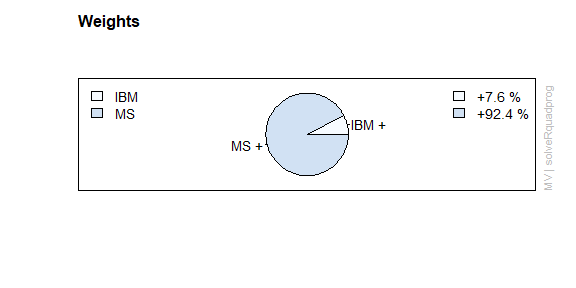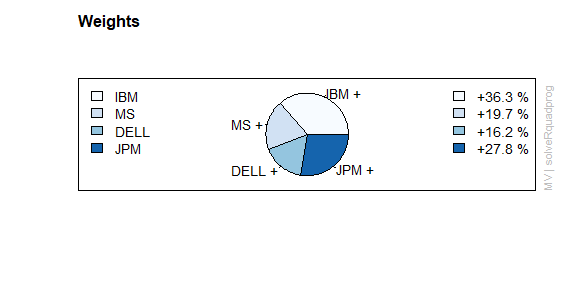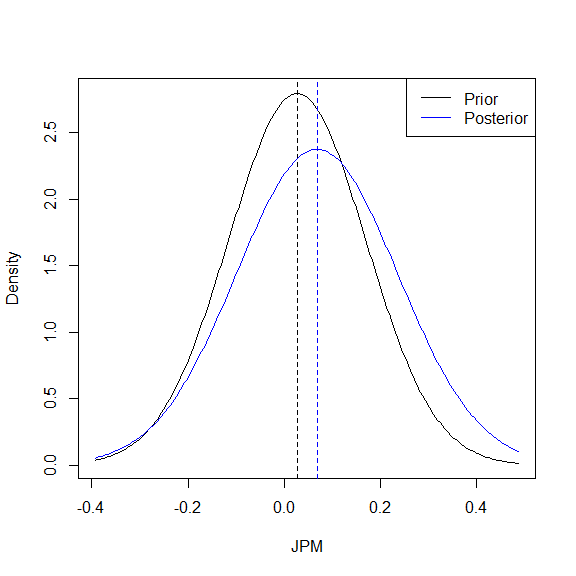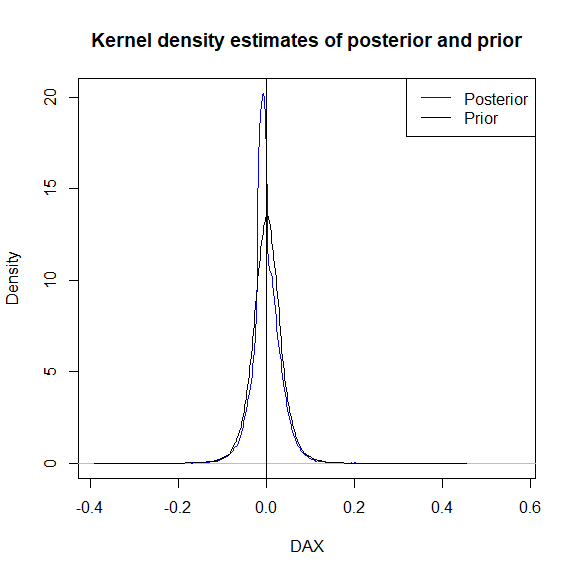# Notes on the BLCOP Package

## Introduction

The BLCOP package is an implementation of the Black-Litterman and copula opinion pooling frameworks. This vignette gives an overview of these two opinion-blending methods, briefly shows how they are implemented in this package, and closes with a short discussion of how the package may evolve in the future (any feedback would be greatly appreciated).

## Overview of the Black-Litterman model

The Black-Litterman model was devised in 1992 by Fisher Black and Robert Litterman. Their goal was to create a systematic method of specifying and then incorporating analyst/portfolio manager views into the estimation of market parameters. Let $$A = \{a_1, a_2, ...,. a_n \}$$ be a set of random variables representing the returns of $$n$$ assets. In the BL approach, the joint distribution of $$A$$ is taken to be multivariate normal, i.e. $$A \sim N(\mu, \Sigma)$$. The problem they then addressed was that of incorporating an analyst’s views into the estimation of the market mean $$\mu$$1

Suppose that we take $$\mu$$ itself to be a random variable which is itself normally distributed, and moreover that its dispersion is proportional to that of the market.
Then $\mu \sim N(\pi, \tau \Sigma),$ and $$\pi$$ is some underlying parameter which can be determined by the analyst using some established procedure. Black and Litterman argued from equilibrium considerations that this should be obtained from the intercepts of the capital-asset pricing model.

Next, the analyst forms subjective views on the actual mean of the returns for the holding period. This is the part of the model that allows the analyst/portfolio manager to include his or her views. BL proposed that views should be made on linear combinations (i.e. portfolios) of the asset return variable means $$\mu$$. Each view would take the form of a “mean plus error” . Thus for example, a typical view would look as follows: $p_{i1} \mu_1 + p_{i2} \mu_2 + ... + p_{in} \mu_n = q_i + \epsilon_i,$ where $$\epsilon_i \sim N(0, \sigma_i^2)$$. The standard deviations $$\sigma_i^2$$ of each view could be taken as controlling the confidence in each view. Collecting these views into a matrix we will call the “pick” matrix, we obtain the “general” view specification $P \mu \sim N(\mu, \Omega).$ $$\Omega$$ is the diagonal matrix $$diag(\sigma_1^2, \sigma_2^2, ..., \sigma_2^n)$$. It can be shown (c.f. (Meucci, n.d.c), p.5 and appendix), based on Bayes’ Law, that the posterior distribution of the market mean conditional on these views is $\mu |_{q; \Omega} \sim N(\mu_{BL}, \Sigma_{BL}^{\mu})$ where \begin{aligned} \mu_{BL} &= ((\tau \Sigma)^{-1} + P^{T} \Omega^{-1} P ) ^{-1} ((\tau \Sigma)^{-1} \pi + P^{T} \Omega^{-1} q) \\ \Sigma_{BL}^{\mu} &= ((\tau \Sigma)^{-1} + P^{T} \Omega^{-1} P)^{-1} \end{aligned}

We can then obtain the posterior distribution of the market by taking $$A|_{q, \Omega} = \mu|_{q, \Omega} + Z$$, and $$Z \sim N(0, \Sigma)$$ is independent of $$\mu$$. One then obtains that $$E[A] = \mu_{BL}$$ and $$\Sigma_{BL} = \Sigma + \Sigma_{BL}^{\mu}$$ ((Meucci, n.d.c), p. 5). Let us now see how these ideas are implemented in the BLCOP package.

## Using the Black-Litterman model in BLCOP

The implementation of the Black-Litterman model in BLCOP is based on objects that represent views on the market and objects that represent the posterior distribution of the market after blending the views. We will illustrate this with a simple example. Suppose that an analyst wishes to form views on 6 stocks, 2 of which are technology stocks and the other 4 of which are from the financial sector. Initially, she believes that the average of the 2 tech stocks will outperform one of the financial stocks, say $$\frac{1}{2}( \textrm{DELL} + \textrm{IBM}) - \textrm{MS} \sim N(0.06, 0.01)$$. We will create a BLViews class object with the BLViews() constructor function. Its arguments are the “pick” matrix, a vector of confidences, the vector “q”, and the the names of the assets in one’s “universe”. Please note that the following examples may require the suggested {fPortfolio} and {mnormt} packages.

library(fPortfolio)
library(BLCOP)
library(mnormt)
pickMatrix <- matrix(c(1/2, -1, 1/2, rep(0, 3)), nrow = 1, ncol = 6 )
views <- BLViews(P = pickMatrix, q = 0.06,confidences =  100,
assetNames = colnames(monthlyReturns))
views
#> 1 : 0.5*IBM+-1*MS+0.5*DELL=0.06  + eps. Confidence: 100

Next, we need to determine the “prior” distribution of these assets. The analyst may for instance decide to set these means to 0, and then calculate the variance-covariance matrix of these through some standard estimation procedure (e.g. exponentially weighted moving average). Here we use cov.mve() from the {MASS} package.

priorMeans <- rep(0, 6)
priorVarcov <- MASS::cov.mve(monthlyReturns)$cov We can now calculate the posterior market distribution using the posteriorEst(). This takes as parameters the view object, the prior covariance and mean, and “tau.”2 The procedure for setting $$\tau$$ is the subject of some controversy in the literature, but here we shall set it to $$1/2$$. marketPosterior <- posteriorEst(views = views, sigma = priorVarcov, mu = priorMeans, tau = 1/2) marketPosterior #> Prior means: #> IBM MS DELL C JPM BAC #> 0 0 0 0 0 0 #> Posterior means: #> IBM MS DELL C JPM #> 0.0093803589 -0.0081392850 0.0180135557 0.0004158929 -0.0050501865 #> BAC #> -0.0026838802 #> Posterior covariance: #> IBM MS DELL C JPM BAC #> IBM 0.01984881 0.010418973 0.013947613 0.010816285 0.010438039 0.005352560 #> MS 0.01041897 0.017997441 0.014331908 0.009602281 0.014598661 0.007356254 #> DELL 0.01394761 0.014331908 0.039600997 0.008962812 0.011782713 0.005652306 #> C 0.01081628 0.009602281 0.008962812 0.010595394 0.009969597 0.006124685 #> JPM 0.01043804 0.014598661 0.011782713 0.009969597 0.019432171 0.008191540 #> BAC 0.00535256 0.007356254 0.005652306 0.006124685 0.008191540 0.007810236 Now suppose that we wish to add another view, this time on the average of the four financial stocks. This can be done conveniently with addBLViews() as in the following example: finViews <- matrix(ncol = 4, nrow = 1, dimnames = list(NULL, c("C","JPM","BAC","MS"))) finViews[,1:4] <- rep(1/4,4) views <- addBLViews(finViews, 0.15, 90, views) views #> 1 : 0.5*IBM+-1*MS+0.5*DELL=0.06 + eps. Confidence: 100 #> 2 : 0.25*MS+0.25*C+0.25*JPM+0.25*BAC=0.15 + eps. Confidence: 90 We will now recompute the posterior, but this time using the captial asset pricing model to compute the “prior” means. Rather than manually computing these, it is convenient to use the BLPosterior() wrapper function. It will compute these “alphas”, as well as the variance-covariance matrix of a returns series, and will then call poseriorEst() automatically. marketPosterior <- BLPosterior(as.matrix(monthlyReturns), views, tau = 1/2, marketIndex = as.matrix(sp500Returns),riskFree = as.matrix(US13wTB)) marketPosterior #> Prior means: #> IBM MS DELL C JPM BAC #> 0.020883598 0.059548398 0.017010062 0.014492325 0.027365230 0.002829908 #> Posterior means: #> IBM MS DELL C JPM BAC #> 0.06344562 0.07195806 0.07777653 0.04030821 0.06884519 0.02592776 #> Posterior covariance: #> IBM MS DELL C JPM BAC #> IBM 0.021334221 0.010575532 0.012465444 0.008518356 0.010605748 0.005281807 #> MS 0.010575532 0.031231768 0.017034827 0.012704758 0.014532900 0.008023646 #> DELL 0.012465444 0.017034827 0.047250599 0.007386821 0.009352949 0.005086150 #> C 0.008518356 0.012704758 0.007386821 0.016267422 0.010968240 0.006365457 #> JPM 0.010605748 0.014532900 0.009352949 0.010968240 0.028181136 0.011716834 #> BAC 0.005281807 0.008023646 0.005086150 0.006365457 0.011716834 0.011199343 Both BLPosterior() and posteriorEst() have a kappa parameter which may be used to replace the matrix $$\Omega$$ of confidences in the posterior calculation. If it is greater than 0, then $$\Omega$$ is set to $$\kappa P^{T} \Sigma P$$ rather than $$diag(\sigma_1^2, \sigma_2^2, ..., \sigma_2^n)$$. This choice of $$\Omega$$ is suggested by several authors, and it leads to the confidences being determined by volatilities of the asset returns. A user may also be interested in comparing allocations that are optimal under the prior and posterior distributions. The {fPortfolio} package of the Rmetrics project ((Rmetrics Core Team, n.d.)), for example, has a rich set of functionality available for portfolio optimization. The helper function optimalPortfolios.fPort() was created to wrap these functions for exploratory purposes. optPorts <- optimalPortfolios.fPort(marketPosterior, optimizer = "tangencyPortfolio") optPorts #>$priorOptimPortfolio
#>
#> Title:
#>  MV Tangency Portfolio
#>  Estimator:         getPriorEstim
#>  Optimize:          minRisk
#>  Constraints:       LongOnly
#>
#> Portfolio Weights:
#>    IBM     MS   DELL      C    JPM    BAC
#> 0.0765 0.9235 0.0000 0.0000 0.0000 0.0000
#>
#> Covariance Risk Budgets:
#>  IBM   MS DELL    C  JPM  BAC
#>
#>
#> Target Returns and Risks:
#>   mean     mu    Cov  Sigma   CVaR    VaR
#> 0.0000 0.0566        0.1460 0.0000 0.0000
#>
#> Description:
#>  Mon Jan 25 15:30:54 2021 by user: jrussell
#>
#> $posteriorOptimPortfolio #> #> Title: #> MV Tangency Portfolio #> Estimator: getPosteriorEstim #> Solver: solveRquadprog #> Optimize: minRisk #> Constraints: LongOnly #> #> Portfolio Weights: #> IBM MS DELL C JPM BAC #> 0.3633 0.1966 0.1622 0.0000 0.2779 0.0000 #> #> Covariance Risk Budgets: #> IBM MS DELL C JPM BAC #> #> #> Target Returns and Risks: #> mean mu Cov Sigma CVaR VaR #> 0.0000 0.0689 0.1268 0.0000 0.0000 #> #> Description: #> Mon Jan 25 15:30:54 2021 by user: jrussell #> #> attr(,"class") #>  "BLOptimPortfolios" weightsPie(optPorts$priorOptimPortfolio)weightsPie(optPorts$posteriorOptimPortfolio)Additional parameters may be passed into function to control the optimization process. Users are referred to the {fPortfolio} package documentation for details. optPorts2 <- optimalPortfolios.fPort(marketPosterior, constraints = "minW[1:6]=0.1", optimizer = "minriskPortfolio") optPorts2 #>$priorOptimPortfolio
#>
#> Title:
#>  MV Minimum Risk Portfolio
#>  Estimator:         getPriorEstim
#>  Optimize:          minRisk
#>  Constraints:       minW
#>
#> Portfolio Weights:
#>    IBM     MS   DELL      C    JPM    BAC
#> 0.1137 0.1000 0.1000 0.1098 0.1000 0.4764
#>
#> Covariance Risk Budgets:
#>  IBM   MS DELL    C  JPM  BAC
#>
#>
#> Target Returns and Risks:
#>   mean     mu    Cov  Sigma   CVaR    VaR
#> 0.0000 0.0157        0.0864 0.0000 0.0000
#>
#> Description:
#>  Mon Jan 25 15:30:54 2021 by user: jrussell
#>
#> $posteriorOptimPortfolio #> #> Title: #> MV Minimum Risk Portfolio #> Estimator: getPosteriorEstim #> Solver: solveRquadprog #> Optimize: minRisk #> Constraints: minW #> #> Portfolio Weights: #> IBM MS DELL C JPM BAC #> 0.1000 0.1000 0.1000 0.1326 0.1000 0.4674 #> #> Covariance Risk Budgets: #> IBM MS DELL C JPM BAC #> #> #> Target Returns and Risks: #> mean mu Cov Sigma CVaR VaR #> 0.0000 0.0457 0.1008 0.0000 0.0000 #> #> Description: #> Mon Jan 25 15:30:54 2021 by user: jrussell #> #> attr(,"class") #>  "BLOptimPortfolios" Finally, density plots of marginal prior and posterior distributions can be generated with densityPlots(). As we will see in the next section, this gives more interesting results when used with copula opinion pooling. densityPlots(marketPosterior, assetsSel = "JPM")## Overview of Copula Opinion Pooling Copula opinion pooling is an alternative way to blend analyst views on market distributions that was developed by Attilio Meucci towards the end of 2005. It is similar to the Black-Litterman model in that it also uses a “pick” matrix to formulate views. However it has several advantages including the following: • Views are made on realizations of the market, not on market parameters as in the original formulation of BL • The joint distribution of the market can be any multivariate distribution • Views are not restricted to the normal distribution • The parameters in the model have clearer meanings • The model can easily be generalized to incorporate the views of multiple analysts Nevertheless, all of this comes at a price. We can no longer use closed-form expressions for calculating the posterior distribution of the market and hence must rely on simulation instead. Before proceeding to the implementation however let us look at the theory. Readers are referred to (Meucci, n.d.b) for a more detailed discussion. As before, suppose that we have a set of $$n$$ assets whose returns are represented by a set of random variables $$A = \{a_1, a_2, ..., a_n \}$$. As in Black-Litterman, we suppose that $$A$$ has some prior joint distribution whose c.d.f we will denote by $$\Phi_A$$. Denote the marginals of this distribution by $$\phi_i$$. An analyst forms his views on linear combinations of future realizations of the values of $$A$$ by assigning subjective probability distributions to these linear combinations. That is we form views of the form $$p_{i,1} a_1 + p_{i,2} a_2 +... + p_{i,n} a_n \sim \theta_i$$, where $$\theta_i$$ is some distribution. Denote the pick matrix formed by all of these views by $$P$$ once again. Now, since we have assigned some prior distribution $$\Phi_A$$ to these assets, it follows that actually the product $$V = PA$$ inherits a distribution as well, say $v_i = p_{i,1} a_1 + p_{i,2} a_2 +... + p_{i,n}a_n \sim \theta_i'$. In general $$\theta_i \neq \theta_i'$$ unless one’s views are identical to the market prior. Thus we must somehow resolve this contradiction. A straightforward way of doing this is to take the weighted sum of the two marginal c.d.fs, so i.e. $$\hat{\theta_i} = \tau_i \theta_i + (1 - \tau_i) \theta_i'$$, and $$\tau_i \in [0,1]$$ is a parameter representing our confidence in our subjective views. This is the actual marginal distribution that will be used to determine the market posterior. The market posterior is actually determined by setting the marginals of distributions of $$V$$ to $$\hat{\theta_i}$$, while using a copula to keep the dependence structure of $$V$$ intact. Let $$V = (v_1, v_2, ..., v_k)$$, where $$k$$ is the number of views that the analyst has formed. Then $$v_i \sim \theta_i'$$. Let $$C$$ be the copula of $$V$$ so that $$C$$ is the joint distribution of $(\theta_1'(v_1), \theta_2'(v_2), ..., \theta_k'(v_k)) = (C_1, C_2, ..., C_k)$ if we now take the $$\theta_i'$$ to be cumulative density functions. Next set $$\hat{V}$$ as the random variable with the joint distribution$(^{-1} (C_1), ^{-1} (C_2), …, ^{-1} (C_k)) $. The posterior market distribution is obtained by rotating $$\hat{V}$$ back into market coordinates using the orthogonal complement of $$P$$. See (Meucci, n.d.b), p.5 for details. ## COP in BLCOP Let us now work through a brief example to see how these ideas are implemented in the BLCOP package. First, one again works with objects that hold the view specification, which in the COP case are of class . These can again be created with a constructor function of the same name. However a significant difference is the use of mvdistribution and distribution class objects to specify the prior distribution and view distributions respectively. We will show the use of these in the following example, which is based on the example used in (Meucci, n.d.b), p.9. Suppose that we wish to invest in 4 market indices (S&P500, FTSE, CAC and DAX). Meucci suggests a multivariate Student-t distribution with $$\nu = 5$$ degrees of freedom and dispersion matrix given by: $10^{-3} \left( \begin{array}{cccc} .376 & .253 & .333 & .397 \\ . &.360 & .360 & .396 \\ . & . & .600 & .578 \\ . & . & . & .775 \end{array} \right).$ He then sets$= w_{eq} \$ where $$w_{eq}$$ is the relative capitilization of the 4 indices and $$\delta = 2.5$$. For simplicity we will simply take $$w_{eq} = (1/4, 1/4, 1/4, 1/4)$$.

dispersion <- c(.376,.253,.360,.333,.360,.600,.397,.396,.578,.775) / 1000
sigma <- BLCOP:::.symmetricMatrix(dispersion, dim = 4)
caps <- rep(1/4, 4)
mu <- 2.5 * sigma %*% caps
dim(mu) <- NULL
marketDistribution <- mvdistribution("mt", mean = mu, S = sigma, df = 5 )
#> Warning in mvdistribution("mt", mean = mu, S = sigma, df = 5): Some functions
#> associated to this distribution could not be found
class(marketDistribution)
#>  "mvdistribution"
#> attr(,"package")
#>  "BLCOP"

The class mvdistribution works with R multivariate probability distribution “suffixes”. mt is the R “name”/“suffix” of the multivariate Student-t as found in the package {mnormt}. That is, the sampling function is given by rmt(), the density by dmt(), and so on. The other parameters are those required by the these functions to fully parameterize the multivariate Student-t. The distribution class works with univariate distributions in a similar way and is used to create the view distributions. We continue with the above example by creating a single view on the DAX.

pick <- matrix(0, ncol = 4, nrow = 1, dimnames = list(NULL, c("SP", "FTSE", "CAC", "DAX")))
pick[1,"DAX"] <- 1
viewDist <- list(distribution("unif", min = -0.02, max = 0))
views <- COPViews(pick, viewDist = viewDist, confidences = 0.2, assetNames = c("SP", "FTSE", "CAC", "DAX"))

As can be seen, the view distributions are given as a list of distribution class objects, and the confidences set the $$tau$$’s described previously. Here we have assigned a $$U(-0.02, 0)$$ distribution to our view with confidence $$0.2$$. Additional views can be added with addCOPViews().

newPick <- matrix(0, 1, 2)
dimnames(newPick) <- list(NULL, c("SP", "FTSE"))
newPick[1,] <- c(1, -1) # add a relative view
views <- addCOPViews(newPick, list(distribution("norm", mean = 0.05, sd = 0.02)), 0.5, views)

The posterior is calculated with COPPosterior(), and the updated marginal distributions can be visualized with densityPlots() once again. The calculation is performed by simulation, based on the ideas described in (Meucci, n.d.a). The simulations of the posterior distribution are stored in the posteriorSims of the class COPResult that is returned by COPPosterior().

marketPosterior <- COPPosterior(marketDistribution, views, numSimulations = 50000)
densityPlots(marketPosterior, assetsSel = 4)## Future developments

While mostly stable, the code is currently in need of some minor cleanup work and refactoring (e.g. pick matrices are referred to as P in some places and pick in others) as well as improvements in the documentation and examples. Attilio Meucci has also very recently proposed an even more general view-blending method which he calls Entropy Pooling and its inclusion would be another obvious extension of this package’s functionality in the longer term.

1. A. Meucci has reformulated the model in terms of forming views directly on market realization rather than the mean, and in my opinion this formulation is considerably clearer. (See Meucci, n.d.c).↩︎

2. An additional parameter called kappa will be discussed shortly↩︎